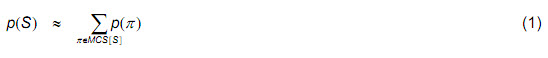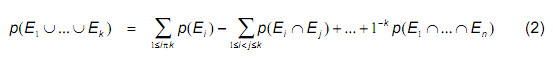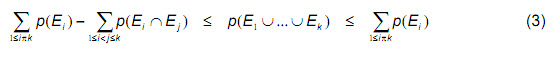# Can We Trust PSA?

Qui a vist Paris et noun Cassis, ren a vist.
If one has seen Paris, but not Cassis, one has seen nothing.
— an old Provencal expression

W. Epstein [ref]ABS Consulting, Koraku Mori, Building, 1-4-14 Koraku Chome, Bunkyo-ku, Tokyo, 112-0004, Japan, sepstein@absconsulting.com [/ref] and A. Rauzy [ref]IML/CNRS, 163, Avenue de Luminy, Case 907, Marseille, 13288 Cedex 9, France, arauzy@iml.univ-mrs.fr[/ref]

Abstract : The Fault Trees/Event Trees method is widely used in industry as the underlying formalism of Probabilistic Risk Assessment. Almost all of the tools available to assess event tree models implement the “classical” assessment technique based on minimal cutsets and the rare event approximation. Binary Decision Diagrams are an alternative approach, but they were up to now limited to medium size models because of the exponential explosion of the memory requirements. We have designed a set of heuristics which make it possible to quantify, by means of BDDs, all of the sequences of a large event tree model coming from the nuclear industry. For one of the first times, it was possible to compare results of the classical approach with those of the BDD approach, i.e. with exact results. This article reports this comparison and shows that the minimal cutsets technique gives wrong results in a significant proportion of cases. Hence, our question in the title of this article.

## 1 Introduction

Katatsuburi
soro-soro nobore
fuji no yama
oh snail
climb Mount Fuji,
but slowly, slowly
— Issa

The Fault Trees/Event Trees method is widely used in industry. Probabilistic Risk Assessment in the nuclear industry relies worldwide almost exclusively on this technique. Several tools are available to assess event tree models. Almost all of them implement what we call the “classical” approach: first, event tree sequences are transformed into Boolean formulae. Then, after possibly applying some rewriting rules, minimal cutsets of these formulae are determined. Finally, various probabilistic measures are assessed from the cutsets (including probabilities and/or frequencies of sequences, importance factors, sensitivity analyzes, …). This approach is broadly accepted. However, it comes with several approximations:

• In order to assess probabilistic quantities from the cutsets, the rare event approximation is applied. Under certain conditions the min-cut upper bound approximation can be used, but only when the boolean equation does not have negation and all basic event probabilities are quite low, at least smaller than 10-2.
• In order to minimize cutsets, and therefore avoiding combinatorial explosion, probability truncation (hereafter referred to as simply truncation) is applied.
• Finally, in order to handle success branches, various recipes more or less mathematically justified are applied.

Since, up to now, all of the assessment tools rely on the same technology (with some variations indeed), it was not possible to verify whether the above approximations are accurate for large real-life models, especially since to compute error bounds, the exact solution is necessary.

In the beginning of the nineties, a new technology was introduced to handle Boolean models: Bryant’s Binary Decision Diagrams (BDD for short) [Bry86,Bry92]. One of the major advantages of the BDD technology is that it provides exact values for probabilistic measures [Rau93,DR00]. It does not need any kind of truncation or approximations. BDDs are however highly memory consuming. Very large models, such as event trees of the nuclear industry, were beyond their reach. Nevertheless, the methodology can be improved by means of suitable variable heuristics and formula rewritings.

Recently, we were given a rather large event tree model (coming from the nuclear industry). We designed a strategy, i.e. a sequence of rewritings, that made it possible to handle all of the 181 sequences of the model within reasonable running times and memory consumptions. For one of the first times, it was possible to compare results of the classical approach with those of the BDD approach, i.e. with exact results. As the epigram to this section intimates, we should not draw definitive conclusions from a single test case. But a single example suffices to ring the alarm bell: the classical approach gives wrong results in a significant proportion of cases. This is true for sequence frequencies and, although to a lesser extent in the problem under study, for component ranking via importance factors.

The remainder of this article is organized as follows. Section 2 is devoted to terminology (Boolean formulae, event trees, …). Sections 3 and 4 present respectively the classical and the BDD approaches. Section 5 gives some insights on the test case we used for this study. Section 6 reports comparative results for the computation of sequence frequencies. Section 7 extends the comparative analysis to importance factors. Section 8 considers briefly the complexity, runtime, and space considerations when trying to solve large problems. Finally, section 9 presents our preliminary conclusions.

## 2 Terminology

Proper notation, the basting that holds the fabric of mathematics in shape, is both the sign and the cause of clear thinking.
— to paraphrase Lynn Truss in EATS, SHOOTS & LEAVES

### 2.1 Boolean Formulae

over a denumerable set of variables and the connectives `and`, `or`, `not`, `k-out-of-n`, and
so on. Their semantics is defined, as usual, by means of the truth tables of
connectives. We denote by `var(F)` the set of variables that occur in the formula F. In
the example to be studied, `F` represents a top event and the variables represent
component failures, or basic events. We use the arithmetic notation for connectives:
`F.G` denotes the formula “F and G” and `F+G` denotes the formula “F or G”. The formula “not F” is denoted either by `-F` or by `F` .

A formula is coherent if it does not contain negations. From a strict mathematical viewpoint, this definition is too restrictive, e.g. `--F` is coherent (assuming `F` is). However, it is sufficient for our purpose.

A literal is either a variable or its negation. A product is a conjunct of literals. It is
sometimes convenient to see products as sets of literals. A minterm of a formula `F` is
a product that contains either positively or negatively each variable of `var(F)`. If n
variables occur in `F`, `2n` minterms can be built over `var(F)`. In other words, minterms one-to-one correspond with truth assignments of variables of `F`. By abuse of notations, we shall write `π(F) = 1` (resp. 0) if the truth assignment that corresponds to the minterm `π` satisfies (resp. falsifies) `F`. We shall say that `π` belongs to `F` when `π(F) = 1`. A formula is always equivalent to the disjunction of its minterms.

Let `π` be a (positive) product and F be a formula. We denote by `πcF` the minterm of `F` built by adding to `π` all the negative literals built over the variables of `F` that do not occur already in `π`. For instance, if `var(F)={a,b,c}` and `π=a`, then `πcF = a.b.c` — We shall omit the subscript when the formula `F` is clear from the context.

Let `π` be a positive product and `F` be a formula. `π` is a cutset of `F` if `πcF` satisfies `F`. A cutset `π` is minimal if no proper subset of `π` is a cutset. We shall denote by `MCS[F]` the set of minimal cutsets of `F`. The reader interested by a more thorough treatment of minimal cutsets should refer to [Rau01].

## 3 The classical approach to assess event trees

Da Vinci was so steeped in his own tradition that each step he took trancended it.
— Scott Buchanan, EMBERS of the WORLD

### 3.1 Principle

By construction, sequences of event trees are mutually exclusive. Therefore, they can be treated separately, at least for what concerns the computation of their probabilities.

The classical approach to assess event trees works as follows.

• First, sequences are compiled as explained above.
• Second, some rewriting is performed on the formula associated with each sequence (e.g. modularization) in order to facilitate their treatment.
• Third, minimal cutsets of each sequence (or group of sequences) are determined. Classical algorithms to compute the minimal cutsets work either top-down (e.g. [FV72, Rau03]) or bottom-up (e.g. [JK98,JHH04]).
• Fourth, probabilities/frequencies of sequences are assessed from the cutsets. More generally, cutsets are used to get various measures of interest such as importance factors of components, sensitivity to variations in basic event probabilities, …

In this process, three kinds of approximations are used:

• Sequences, including success branches, are quantified by means of minimal cutsets (which, by definition, do not embed negations).
• Truncation is applied to limit the process, and therefore reduce the possibility of combinatorial explosion.
• Probabilities are evaluated using one of two first order approximations: the rare event approximation or min-cut upper bound.

In the remainder of this section, we shall discuss the consequences of these three kinds of approximations.

### 3.2 The rare event approximation

Let us assume, for a while, that minimal cutsets represent exactly the sequence. The rare events approximation is used to assess the probability of the sequence. Namely, for a sequence `S` (or more exactly the Boolean formula `S` that represents the sequence), the probability of `S` is assessed as follows.The rare event approximation is actually the first term of the Sylvester-Poincaré development to compute the probability of a union of events:The rare event approximation gives an upper bound of the probability, it is therefore conservative. By computing the second term of the development, one gets a lower bound of the probability (these two values constitute the first pair of so-called BooleBonferroni bounds):When the number of cutsets is large, the computation of more terms is intractable. The rare event approximation gives accurate results when the probabilities of basic events are low. In the presence of relatively high probabilities (say `>10-2`) and/or many minimal cutsets, the approximation is no longer valid. Consider for instance a 3-out-of-6 system `S`, with `p(e)=0.1` for each basic event e. The exact probability of `S` is `0.01585`. The Boole-Bonferroni bounds given by equation (3) are respectively
`0.01009` and `0.02`, a rather rough approximation in both cases. The min-cut upper bound approximation is also no longer valid when relatively high probabilities are present, either from negation or embedding alignment frequencies in the fault trees.

### 3.3 Truncation in minimal cutsets determination

In general, sequences of large event trees admit huge numbers of minimal cutsets. Therefore, only a subset of the latter’s can be considered (the most important ones, in terms of probability, one expects). Algorithms to compute minimal cutsets apply truncation to keep only few thousands cutsets (beyond computations are intractable). The choice of the right truncation value is a result of trade-offs between accuracy of the computation and resource (time and memory) consumption. Expert knowledge about the expected probability of the sequence plays also an important role in that choice.

It remains that, by applying truncation, one gets an optimistic approximation. Moreover, there is no way to ensure that this approximation is accurate. For instance, if we keep a thousand cutsets of probability 10-9 and by the way we ignore a million cutsets of order 10-11, then we underestimate the risk by a factor 10. This problem is largely ignored by most of the practitioners.

[page7]